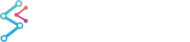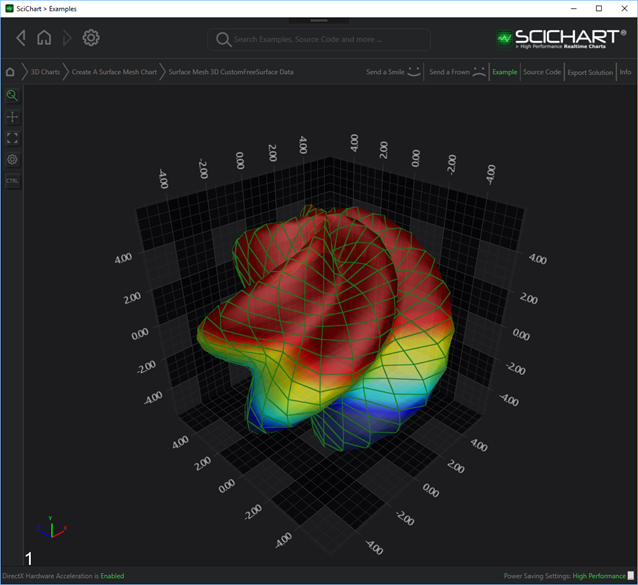﻿ The Custom Free Surface 3D Chart Type | WPF Chart DocumentationSciChart WPF 3D Charts > 3D Chart Types > The Free Surface 3D Chart Types > The Custom Free Surface 3D Chart Type
The Custom Free Surface 3D Chart Type

Examples for the 3D Surface Mesh Chart can be found in the SciChart WPF Examples Suite which can be downloaded from the SciChart Website or our SciChart.WPF.Examples Github Repository.

NEW to SciChart WPF 3D v5.2!

Custom Free Surface 3D Charts are provided by the CustomFreeSurfaceDataSeries3D type.The shape of its surface is defined by a set of user-defined functions, injected in the constructor during the instantiation. This approach allows the surface to obtain any possible shape.

CustomFreeSurfaceDataSeries3D Constructor
Copy Code
```public CustomFreeSurfaceDataSeries3D(int uCount, int vCount,
Func<double, double, double> azimuthalAngleFunction,
Func<double, double, double> polarAngleFunction,
Func<double, double, double, TX> xFunction,
Func<double, double, double, TY> yFunction,
Func<double, double, double, TZ> zFunction,
double uMin = 0.0, double uMax = Math.PI * 2.0,
double vMin = 0.0, double vMax = Math.PI)
```

• Radial Distance Function – is a user-defined function that determines how distance from the origin to the particular point on the surface differs.
• Azimuthal Angle Function – is a user-defined function that determines the azimuthal angle between the particular point and the unit vector of the X-Axis, projected on the ZX plane.
• Polar Angle Function – is a user-defined function that determines inclination (or polar angle) between the particular point and the unit vector of the Y-Axis (Zenith).

The U and V coordinates in intervals [uMin..uMax]  and [vMin..vMax] respectively are passed as the arguments to each of the three functions above.

• X Function - is a user-defined function that determines the position of the particular point on the surface by the X-Axis.
• Y Function - is a user-defined function that determines the position of the particular point on the surface by the Y-Axis.
• Z Function - is a user-defined function that determines the position of the particular point on the surface by the Z-Axis.

The Radial Distance, Polar Angle, and Azimuthal Angle are passed as the arguments to each of the three functions above.

The location of the CustomFreeSurfaceSeries3D is defined by following properties:

• CustomFreeSurfaceSeries3D.OffsetX – a location of the Custom Free Surface by the X-Axis
• CustomFreeSurfaceSeries3D.OffsetY – a location of the Custom Free Surface by the Y-Axis
• CustomFreeSurfaceSeries3D.OffsetZ – a location of the Custom Free Surface by the Z-Axis

## Custom Free Surface Mesh Examples

### Declaring the Custom Free Surface 3D Series that defines a free surface mesh

The following code will create the shape at the top of this article:

```<s3D:SciChart3DSurface x:Name="sciChart" Grid.Column="1" WorldDimensions="200,200,200" BorderThickness="0">
<s3D:SciChart3DSurface.Camera>
<s3D:Camera3D ZoomToFitOnAttach="True"/>
</s3D:SciChart3DSurface.Camera>
<s3D:SciChart3DSurface.RenderableSeries>
<s3D:FreeSurfaceRenderableSeries3D x:Name="customFreeSurfaceRenderableSeries"
DrawMeshAs="SolidWireFrame"
Stroke="#77228B22"
ContourStroke="#77228B22"
StrokeThickness="2.0"
LightingFactor="{Binding ElementName=lightingFactorSlider, Path=Value, Mode=TwoWay}"
Opacity="{Binding ElementName=opacitySlider, Path=Value, Mode=TwoWay}"
MeshColorPalette="{StaticResource HeightColorMap}"
PaletteMinimum="0.0, 5.0, 0.0" PaletteMaximum="0.0, 7.0, 0.0" />
</s3D:SciChart3DSurface.RenderableSeries>
<s3D:SciChart3DSurface.XAxis>
<s3D:NumericAxis3D />
</s3D:SciChart3DSurface.XAxis>
<s3D:SciChart3DSurface.YAxis>
<s3D:NumericAxis3D />
</s3D:SciChart3DSurface.YAxis>
<s3D:SciChart3DSurface.ZAxis>
<s3D:NumericAxis3D />
</s3D:SciChart3DSurface.ZAxis>
</s3D:SciChart3DSurface>
```
```private void OnLoaded(object sender, RoutedEventArgs routedEventArgs)
{
int countU = 30;
int countV = 30;
var meshDataSeries = new CustomFreeSurfaceDataSeries3D<double>(countU, countV,
(u, v) => 5.0 + Math.Sin(5 * (u + v)),
(u, v) => u,
(u, v) => v,
(r, theta, phi) => r * Math.Sin(theta) * Math.Cos(phi),
(r, theta, phi) => r * Math.Cos(theta),
(r, theta, phi) => r * Math.Sin(theta) * Math.Sin(phi))
{
SeriesName = "Custom Free Surface"
};
customFreeSurfaceRenderableSeries.DataSeries = meshDataSeries;
}
```

### Declaring the Custom Free Surface 3D Series that defines the sphere with a radius of 10

To create a perfect sphere with a Radius of 10 using the CustomFreeSurfaceDataSeries3D, replace your code behind with this. Use the same View code as above.

Code Behind
Copy Code
```private void OnLoaded(object sender, RoutedEventArgs routedEventArgs)
{
int countU = 30;
int countV = 30;
var meshDataSeries = new CustomFreeSurfaceDataSeries3D<double>(countU, countV,
(u, v) => u,
(u, v) => v,
(r, theta, phi) => r * Math.Sin(theta) * Math.Cos(phi),
(r, theta, phi) => r * Math.Cos(theta),
(r, theta, phi) => r * Math.Sin(theta) * Math.Sin(phi))
{
SeriesName = "Free Surface Sphere"
};
customFreeSurfaceRenderableSeries.DataSeries = meshDataSeries;
}
```

### Declaring the Custom Free Surface 3D Series that defines the cylinder with a radius of 10 and a height of 40

To create a cylinder with a radius of 10 and height of 40 using the CustomFreeSurfaceDataSeries3D, replace your code behind with this. Use the same View code as above.

Code Behind
Copy Code
```private void OnLoaded(object sender, RoutedEventArgs routedEventArgs)
{
int countU = 30;
int countV = 30;
double halfHeight = 40.0 / 2.0;
var meshDataSeries = new CustomFreeSurfaceDataSeries3D<double>(countU, countV,
(u, v) => 0.0,
(u, v) => u,
(u, v) => v,
(_, theta, phi) => radius * Math.Sin(Math.PI / 2.0) * Math.Cos(phi),
(_, theta, phi) => halfHeight * Math.Cos(theta),
(_, theta, phi) => radius * Math.Sin(Math.PI / 2.0) * Math.Sin(phi))
{
SeriesName = "Free Surface Cylindroid"
};
customFreeSurfaceRenderableSeries.DataSeries = meshDataSeries;
customFreeSurfaceRenderableSeries.DrawBackSide = true;
}
```